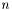#Boost C++ Libraries

...one of the most highly regarded and expertly designed C++ library projects in the world.

## Struct template weighted_variance_impl

boost::accumulators::impl::weighted_variance_impl — Iterative calculation of variance of weighted samples.

## Synopsis

```// In header: <boost/accumulators/statistics/weighted_variance.hpp>

template<typename Sample, typename Weight, typename MeanFeature, typename Tag>
struct weighted_variance_impl {
// types
typedef numeric::functional::multiplies< Sample, Weight >::result_type       weighted_sample;
typedef numeric::functional::average< weighted_sample, Weight >::result_type result_type;

// construct/copy/destruct
template<typename Args> weighted_variance_impl(Args const &);

// public member functions
template<typename Args> void operator()(Args const &) ;
result_type result(dont_care) const;
};```

## Description

Iterative calculation of variance of weighted samples:

Equation 1.43.whereis the sum of theweightsandthe estimate of the mean of the weighted smaples. Note that the sample variance is not defined for.

### `weighted_variance_impl` public construct/copy/destruct

1. `template<typename Args> weighted_variance_impl(Args const & args);`

### `weighted_variance_impl` public member functions

1. `template<typename Args> void operator()(Args const & args) ;`
2. `result_type result(dont_care) const;`